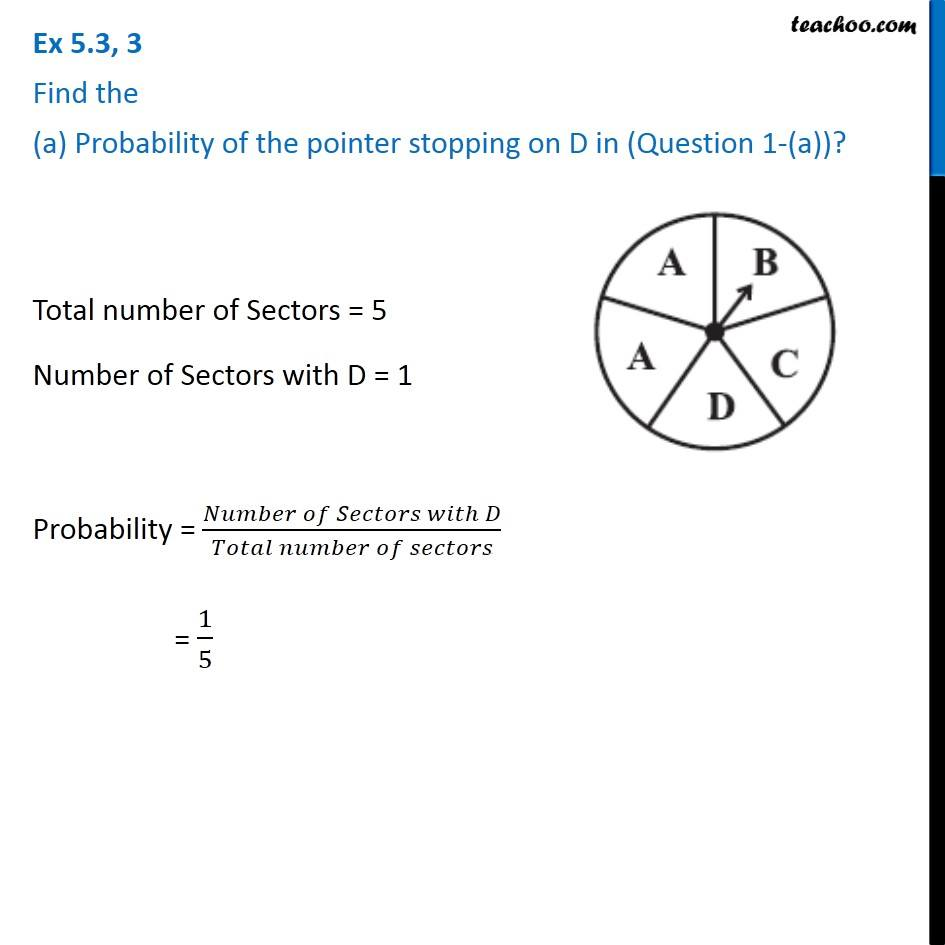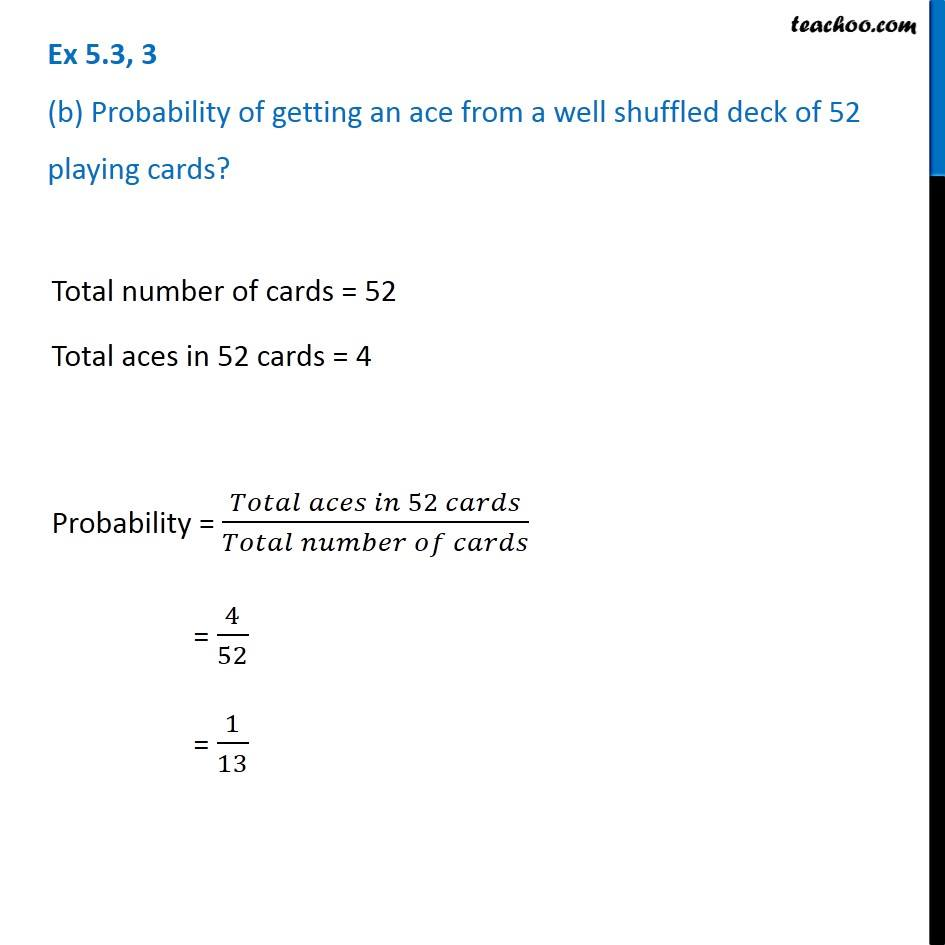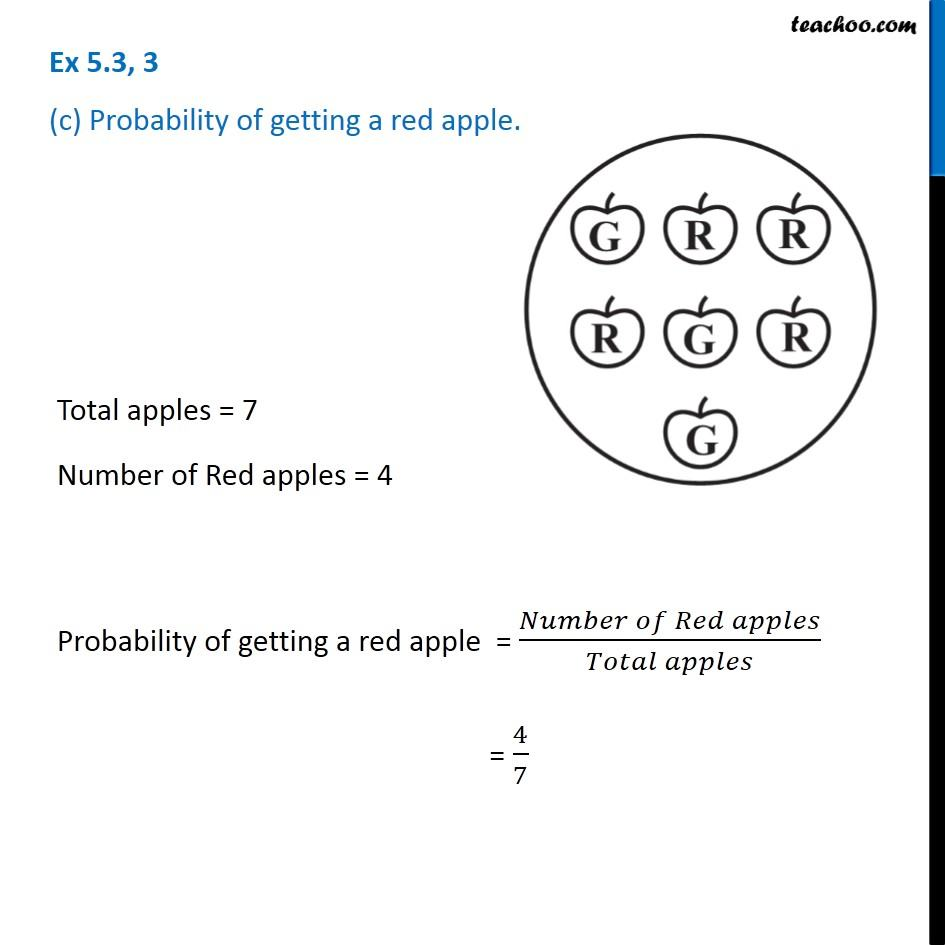Subscribe to our Youtube Channel - https://you.tube/teachoo

1. Chapter 5 Class 8 Data Handling
2. Serial order wise
3. Ex 5.3

Transcript

Ex 5.3, 3 Find the (a) Probability of the pointer stopping on D in (Question 1-(a))? Total number of Sectors = 5 Number of Sectors with D = 1 Probability = (𝑁𝑢𝑚𝑏𝑒𝑟 𝑜𝑓 𝑆𝑒𝑐𝑡𝑜𝑟𝑠 𝑤𝑖𝑡ℎ 𝐷)/(𝑇𝑜𝑡𝑎𝑙 𝑛𝑢𝑚𝑏𝑒𝑟 𝑜𝑓 𝑠𝑒𝑐𝑡𝑜𝑟𝑠) = 1/5 Ex 5.3, 3 (b) Probability of getting an ace from a well shuffled deck of 52 playing cards? Total number of cards = 52 Total aces in 52 cards = 4 Probability = (𝑇𝑜𝑡𝑎𝑙 𝑎𝑐𝑒𝑠 𝑖𝑛 52 𝑐𝑎𝑟𝑑𝑠)/(𝑇𝑜𝑡𝑎𝑙 𝑛𝑢𝑚𝑏𝑒𝑟 𝑜𝑓 𝑐𝑎𝑟𝑑𝑠) = 4/52 = 1/13 Ex 5.3, 3 (c) Probability of getting a red apple. Total apples = 7 Number of Red apples = 4 Probability of getting a red apple = (𝑁𝑢𝑚𝑏𝑒𝑟 𝑜𝑓 𝑅𝑒𝑑 𝑎𝑝𝑝𝑙𝑒𝑠)/(𝑇𝑜𝑡𝑎𝑙 𝑎𝑝𝑝𝑙𝑒𝑠) = 4/7

Ex 5.3